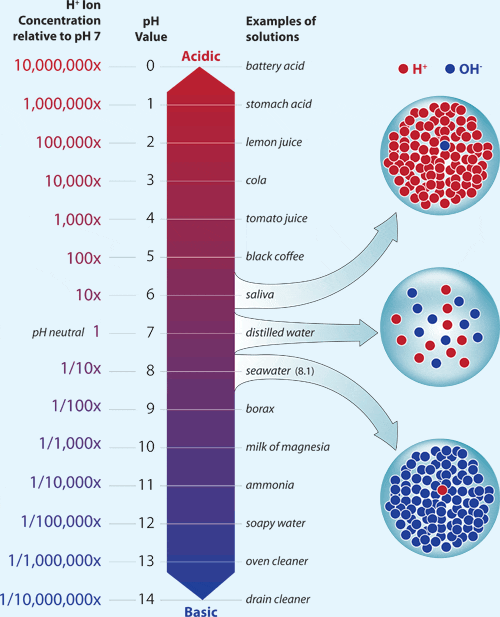The pH Scale

pH is a measure of the concentration of hydrogen ions in a solution. The more hydrogen ions that are present, the more acidic is the solution. The pH scale ranges from zero (very acidic) to 14 (very basic).

A pH of 7 is neutral, a pH less than 7 is acidic, and a pH greater than 7 is basic. For example, the pH of lemon juice is 2 while some antacids have a pH of about 10.

Ocean water is normally slightly basic, with a surface-water pH of about 8.2, but that has declined in recent years to about 8.1. A decrease of 0.1 pH units may not seem like much, but because the pH scale is logarithmic, each unit on the pH scale represents a tenfold change in acidity.

For example, water with a pH of 6 is ten times more acidic than neutral water with a pH of 7, and 100 times more acidic than water with a pH of 8. So a decrease of 0.1 pH units represents a 26 percent increase in the relative acidity of ocean water.(Woods Hole Oceanographic Institution)